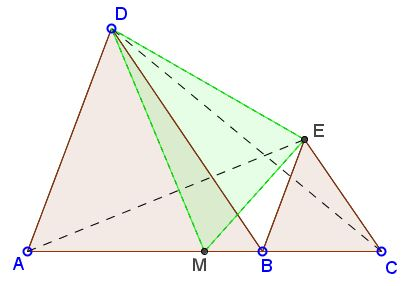# A Lemma of Equal Areas

Bui Quang Tuan came up with a simple but surprisingly useful lemma:

Let $[X]$ denote the area of shape $X.$

Let $A,$ $B,$ $C$ be three collinear points. $D,$ $E$ are two points on one side with respect to line that contains $A,$ $B,$ $C$ and such that $AD\parallel BE$ and $BD\parallel CE.$ $M$ is midpoint of $AC.$ Then $[\Delta DEM] = ([\Delta ABD] + [\Delta BCE])/2.$Observe that if $B$ coincides with $M,$ the claim is rather obvious, for then the three triangles $DEM,$ $ABD,$ and $BCE$ are equal. Interestingly, the equality of the areas holds in a more general case.

17 April 2015, Created with GeoGebra

ProofLet $A,$ $B,$ $C$ be three collinear points. $D,$ $E$ are two points on one side with respect to line that contains $A,$ $B,$ $C$ and such that $AD\parallel BE$ and $BD\parallel CE.$ $M$ is midpoint of $AC.$ Then $[\Delta DEM] = ([\Delta ABD] + [\Delta BCE])/2.$### Proof

In the trapezoid $ABED,$ $[\Delta ABD] = [\Delta AED]$ because the two triangles share a base $AD$ and are in the same parallel lines, meaning that the altitudes to $AD$ from $B$ and $E$ are equal. Similarly, in the trapezoid $BCED,$ $[\Delta BCE] = [\Delta CDE].$ Adding the two identities,

$[\Delta ABD] + [\Delta BCE] = [\Delta AED] + [\Delta CDE].$

Let $h_{a},$ $h_{m},$ $h_{c}$ be the lengths of the perpendiculars to $DE$ from $A,$ $M,$ and $C,$ respectively. $h_{m} = (h_{a} + h_{c})/2.$ On the other hand, $[\Delta AED] = DE\cdot h_{a}/2$ and $[\Delta CDE] = DE\cdot h_{c}/2.$ Therefore,

\begin{align} [\Delta ABD] + [\Delta BCE]&= [\Delta AED] + [\Delta CDE]\\ &= DE\cdot (h_{a} + h_{c})/2\\ &= DE\cdot h_{m}\\ &= 2\cdot [\Delta DEM].\\ \end{align}

### Note

If $h_{b}$ is the altitude from $B$ to $DE,$ then - using similarities - $h_{b}/h_{a} = h_{c}/h_{b},$ from which $[\Delta ADE)\times [\Delta CDE] = [\Delta BDE]^{2}.$ As a consequence of the above, we also have $[\Delta ABD]\times [\Delta BCE] = [\Delta BDE]^{2}.$